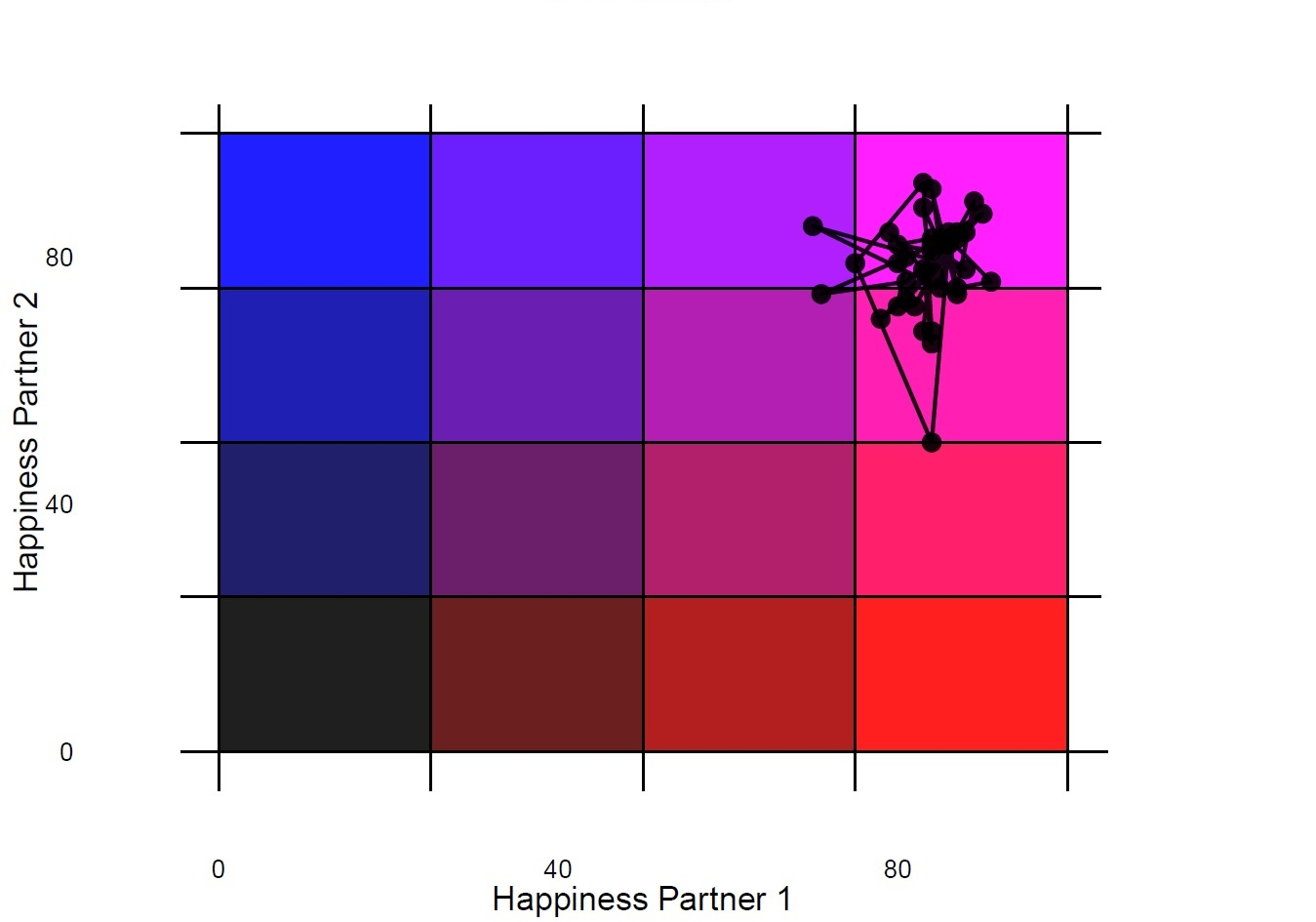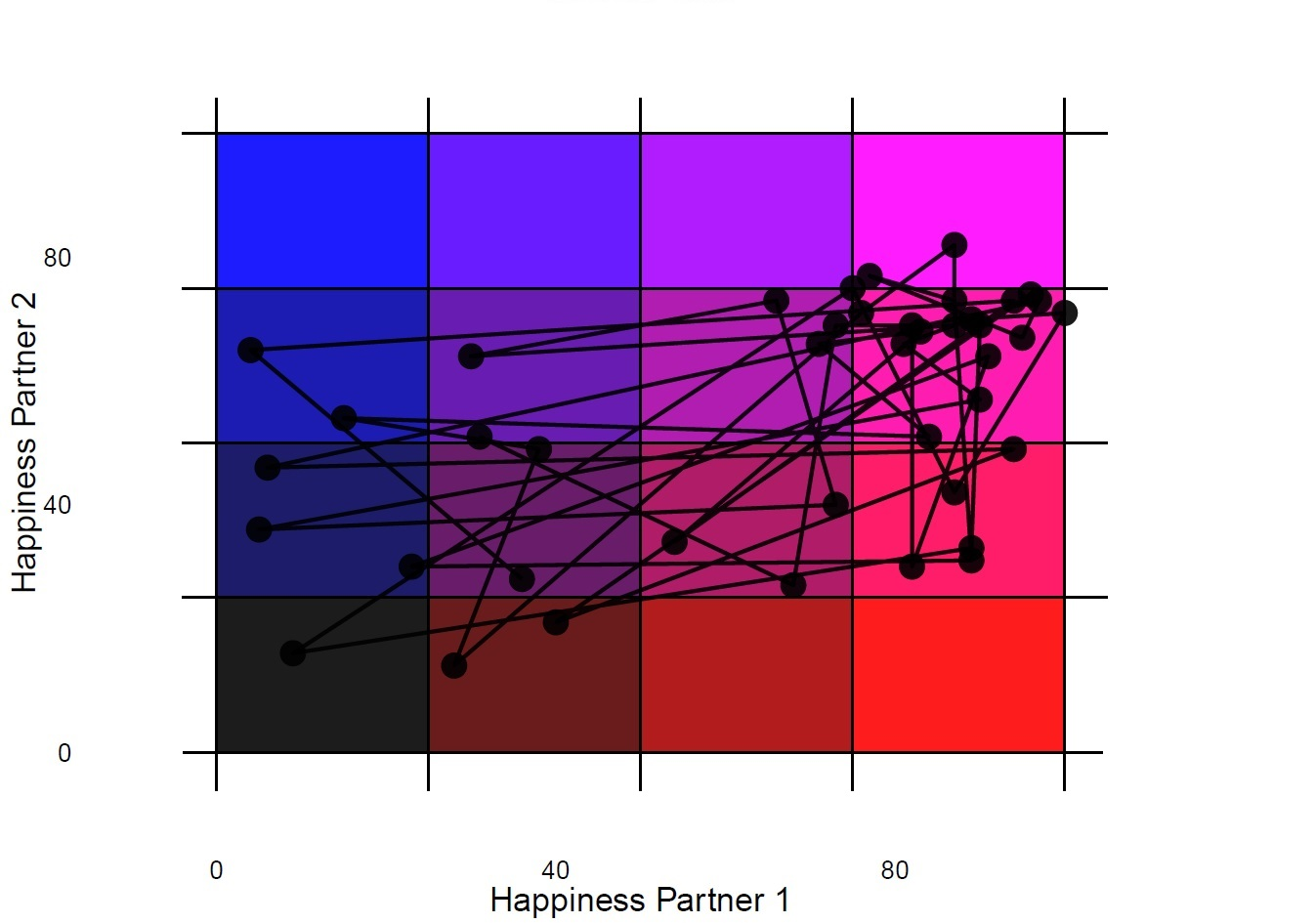## Outline

1. Introduction to Grid-Sequence Analysis.
2. Creating State-Space Grids for Each Dyad.
3. Creating Sequences.
4. Establishing a Cost Matrix and Sequence Analysis.
5. Cluster Determination.
6. Examine Group Differences among Clusters.

## 0. Introduction to Grid-Sequence Analysis.

Grid-sequence analysis is a descriptive technique to capture within-dyad dynamics and allow for between-dyad comparisons. This analytic technique draws from state-space grids, typically used in developmental psychology (Hollenstein, 2013) and sequence analysis, previously used in sociology and biology (Macindoe & Abbott, 2004).

Grid-sequence analysis combines state-space grids and sequence analysis by:

1. Tracking a dyad’s movements across a grid.
2. Converting dyad movements into a univariate sequence.
3. Clustering dyads with similar movements using sequence analysis.

``````#set filepath for repeated measures of happiness data file
filepath <- "https://quantdev.ssri.psu.edu/sites/qdev/files/gridsequence_simulation_data.csv"
``````##   X id time1  outcome1  outcome2
## 1 1  1     1  88.27175 100.00000
## 2 2  1     2  93.70530  88.49227
## 3 3  1     3  97.49972  91.96082
## 4 4  1     4 100.00000 100.00000
## 5 5  1     5  96.65027  84.73661
## 6 6  1     6  96.44185 100.00000``````
``````#setwd("~/Desktop")
#set filepath for person-level data (e.g., relationship satisfaction and current health)
filepath1 <- "https://quantdev.ssri.psu.edu/sites/qdev/files/gridsequence_simulation_descriptives.csv"
``````##   id member  rel_sat   health
## 1  1      1 1.388314 2.747397
## 2  1      2 1.895276 2.032986
## 3  2      1 1.074569 1.548543
## 4  2      2 1.022392 1.843375
## 5  3      1 1.052483 1.883714
## 6  3      2 2.006875 3.357317``````
``````#delete first column of each data set (1, 2, ..., n labeling in Excel sheet)

data <- NULL
``````##   id time1  outcome1  outcome2
## 1  1     1  88.27175 100.00000
## 2  1     2  93.70530  88.49227
## 3  1     3  97.49972  91.96082
## 4  1     4 100.00000 100.00000
## 5  1     5  96.65027  84.73661
## 6  1     6  96.44185 100.00000``````
``````#loading libraries
library(cluster)
library(ggplot2)
library(reshape)
library(stats)
library(TraMineR)
library(TraMineRextras)``````

Depending on the format of your data set, some data management may be necessary. The final product should be two data sets:

1. “data” contains repeated measures of the variable of interest (in this case, happiness). There should be a column that contains a couple-level ID variable, a column the contains a continuous measure of time (in this case, 1-42, given that we measured happiness 42 times over a week), and columns for each member of the dyad’s responses to our happiness item.

2. “data1” contains person-level, time-invariant variables. These are the variables in which you will test between-group differences in Step 5 of grid-sequence analysis. This data file should include a column for couple-level ID, a column that distinguishes each member of the dyad (in this case labeled “member”), and columns with the between-person/dyad variables of interests (such as relationship satisfaction or subjective health).

## 1. Creating State-Space Grids for Each Dyad.

We begin creating our state-space grid by setting up cut points. In this example, we create a 4 x4 (16 cell) grid, and use three cut points (at intervals of 25 because our measure of happiness is on a 0-100 scale). Our cut points are only for the sake of demonstration, but these divisions can vary depending on your research question or underlying theory.

``````#Creating cut points
data\$cut1 <- 25
data\$cut2 <- 50
data\$cut3 <- 75``````

Next we use these cut points to label the cells on the grid with letters of the English alphabet and create a new variable that contains these letters (labeled as “happy” in our data set “data”). We set the x-axis to be dyad member 1 (who is associated with “outcome1”) and the y-axis to be dyad member 2 (who is associated with “outcome2”). In this case, we label the upper-left hand corner with “A” and the lower-right hand corner with “P,” filling in alphabetically along the way.

``````#Lettering each cell of the grid
data\$happy[data\$outcome1 <= data\$cut1 & data\$outcome2 > data\$cut3] <- "A"

data\$happy[data\$outcome1 > data\$cut1 & data\$outcome1 <= data\$cut2 & data\$outcome2 > data\$cut3] <- "B"

data\$happy[data\$outcome1 > data\$cut2 & data\$outcome1 <= data\$cut3 & data\$outcome2 > data\$cut3] <- "C"

data\$happy[data\$outcome1 > data\$cut3 & data\$outcome2 > data\$cut3] <- "D"

data\$happy[data\$outcome1 <= data\$cut1 & data\$outcome2 > data\$cut2 & data\$outcome2 <= data\$cut3] <- "E"

data\$happy[data\$outcome1 > data\$cut1 & data\$outcome1 <= data\$cut2 & data\$outcome2 > data\$cut2 & data\$outcome2 <= data\$cut3] <- "F"

data\$happy[data\$outcome1 > data\$cut2 & data\$outcome1 <= data\$cut3 & data\$outcome2 > data\$cut2 & data\$outcome2 <= data\$cut3] <- "G"

data\$happy[data\$outcome1 > data\$cut3 & data\$outcome2 > data\$cut2 & data\$outcome2 <= data\$cut3] <- "H"

data\$happy[data\$outcome1 <= data\$cut1 & data\$outcome2 > data\$cut1 & data\$outcome2 <= data\$cut2] <- "I"

data\$happy[data\$outcome1 > data\$cut1 & data\$outcome1 <= data\$cut2 & data\$outcome2 > data\$cut1 & data\$outcome2 <= data\$cut2] <- "J"

data\$happy[data\$outcome1 > data\$cut2 & data\$outcome1 <= data\$cut3 & data\$outcome2 > data\$cut1 & data\$outcome2 <= data\$cut2] <- "K"

data\$happy[data\$outcome1 > data\$cut3 & data\$outcome2 > data\$cut1 & data\$outcome2 <= data\$cut2] <- "L"

data\$happy[data\$outcome1 <= data\$cut1 & data\$outcome2 <= data\$cut1] <- "M"

data\$happy[data\$outcome1 > data\$cut1 & data\$outcome1 <= data\$cut2 & data\$outcome2 <= data\$cut1] <- "N"

data\$happy[data\$outcome1 > data\$cut2 & data\$outcome1 <= data\$cut3 & data\$outcome2 <= data\$cut1] <- "O"

data\$happy[data\$outcome1 > data\$cut3 & data\$outcome2 <= data\$cut1] <- "P"``````

A quick check to see what the repeated-measures data looks like:

``head(data)``
``````##   id time1  outcome1  outcome2 cut1 cut2 cut3 happy
## 1  1     1  88.27175 100.00000   25   50   75     D
## 2  1     2  93.70530  88.49227   25   50   75     D
## 3  1     3  97.49972  91.96082   25   50   75     D
## 4  1     4 100.00000 100.00000   25   50   75     D
## 5  1     5  96.65027  84.73661   25   50   75     D
## 6  1     6  96.44185 100.00000   25   50   75     D``````

It is useful to plot each dyad’s movements across the state-space grid to obtain a greater understanding of the data. I’ve attached plots of two different dyad’s (one with low variability and one with high variability).Below is code for a loop to obtain a PDF of each dyad’s state space grid.

``````all_ids <- unique(data\$id)

# I'm going to remove the NAs from all_ids

all_ids <- na.omit(all_ids)

cut1 <- 25
cut2 <- 50
cut3 <- 75

# open the pdf file
pdf('State Space Grid Simulation.pdf', width = 10, height = 7)

for(x in 1:length(all_ids)){
data_loop_subset <- subset(data, id == dyad_id)

#let's remove the missing data

data_loop_noNA <- na.omit(data_loop_subset)

setwd("~/Desktop")

# At this point we need to check if there is actually data for the individual and only plot points where there are data for both members of the dyad at each time point.

if(nrow(data_loop_noNA) == 0){}

if(nrow(data_loop_noNA) > 0){

plot_title = paste('Couple ID = ', unique(data_loop_noNA\$id), sep = '') #adding a dyad ID label at the top of each plot
grid_plot <-

ggplot(data = data_loop_noNA, aes(x = outcome1, y = outcome2)) +
xlim(-5,105) + #giving a little extra room (the scale is 0-100) so points of the edge of the scale will show up easily
ylim(-5,105) + #giving a little extra room (the scale is 0-100) so points of the edge of the scale will show up easily
ylab('Happiness Partner 2') + #y-axis label
xlab('Happiness Partner 1') + #x-axis label
geom_rect(xmin=0,xmax=cut1,ymin=0,ymax=cut1, fill="#000000", alpha=.05) + #the following geom_rect lines of code are assigning colors to each cell of the grid and adjusting their transparency (with the alpha = command)
geom_rect(xmin=0,xmax=cut1,ymin=cut1,ymax=cut2, fill="#000054", alpha=.05) +
geom_rect(xmin=0,xmax=cut1,ymin=cut2,ymax=cut3, fill="#0000A8", alpha=.05) +
geom_rect(xmin=0,xmax=cut1,ymin=cut3,ymax=100, fill="#0000FC", alpha=.05) +
geom_rect(xmin=cut1,xmax=cut2,ymin=0,ymax=cut1, fill="#540000", alpha=.05) +
geom_rect(xmin=cut1,xmax=cut2,ymin=cut1,ymax=cut2, fill="#540054", alpha=.05) +
geom_rect(xmin=cut1,xmax=cut2,ymin=cut2,ymax=cut3, fill="#5400A8", alpha=.05) +
geom_rect(xmin=cut1,xmax=cut2,ymin=cut3,ymax=100, fill="#5400FC", alpha=.05) +
geom_rect(xmin=cut2,xmax=cut3,ymin=0,ymax=cut1, fill="#A80000", alpha=.05) +
geom_rect(xmin=cut2,xmax=cut3,ymin=cut1,ymax=cut2, fill="#A80054", alpha=.05) +
geom_rect(xmin=cut2,xmax=cut3,ymin=cut2,ymax=cut3, fill="#A800A8", alpha=.05) +
geom_rect(xmin=cut2,xmax=cut3,ymin=cut3,ymax=100, fill="#A800FC", alpha=.05) +
geom_rect(xmin=cut3,xmax=100,ymin=0,ymax=cut1, fill="#FC0000", alpha=.05) +
geom_rect(xmin=cut3,xmax=100,ymin=cut1,ymax=cut2, fill="#FC0054", alpha=.05) +
geom_rect(xmin=cut3,xmax=100,ymin=cut2,ymax=cut3, fill="#FC00A8", alpha=.05) +
geom_rect(xmin=cut3,xmax=100,ymin=cut3,ymax=100, fill="#FC00FC", alpha=.05) +
theme(
axis.text = element_text(size = 14, color = 'black'),
axis.title = element_text(size = 18),
panel.grid.major = element_line(colour = "white"),
panel.grid.minor = element_blank(),
panel.background = element_blank(),
axis.ticks = element_blank()
) + #adjusting size of text and titles in plot
geom_vline(xintercept = c(0,25,50,75,100)) + #adding vertical lines to distinguish cells of grid
geom_hline(yintercept = c(0,25,50,75,100)) + #adding horizontal lines to distinguish cells of grid
geom_point(colour= "white") + #setting points on plot to white
geom_path(colour = "white")  + #setting path connecting points to the color white
print(grid_plot)
}
}

dev.off()``````

## 2. Creating Sequences.

In this step, we re-format the data from long to wide, create an “alphabet” that consists of the letters in our grid, and extract the sequence of cells visited. Helpful comments are included within the code.

``````#subsetting out the data we need
data_sub <- data[ ,c("id", "time1", "happy")]

#Reformatting data: long to wide.
data_wide <- reshape(data=data_sub,
timevar=c("time1"),            #time variable
idvar= c("id"),                #id variable
v.names=c("happy"),            #repeated measures variable
direction="wide", sep=".")

``````##     id happy.1 happy.2 happy.3 happy.4 happy.5 happy.6 happy.7 happy.8
## 1    1       D       D       D       D       D       D       D       D
## 43   2       H       D       D       D       D       H       D       D
## 85   3       O       H       H       G       D       L       G       L
## 127  4       I       E       J       N       F       M       K       N
## 169  5       D       C       D       D       C       D       C       C
## 211  6       D       H       H       H       D       D       H       H
##     happy.9 happy.10 happy.11 happy.12 happy.13 happy.14 happy.15 happy.16
## 1         D        D        D        D        D        D        H        D
## 43        D        H        D        H        D        H        H        D
## 85        L        H        G        C        B        D        J        G
## 127       N        M        M        N        N        J        E        J
## 169       C        D        C        C        B        C        C        C
## 211       H        H        H        H        H        D        H        D
##     happy.17 happy.18 happy.19 happy.20 happy.21 happy.22 happy.23
## 1          D        D        D        D        D        D        D
## 43         D        D        D        D        D        D        D
## 85         E        L        G        J        O        O        H
## 127        I        M        N        M        J        A        N
## 169        B        D        C        B        C        D        D
## 211        D        D        H        H        D        D        D
##     happy.24 happy.25 happy.26 happy.27 happy.28 happy.29 happy.30
## 1          D        D        D        D        H        H        D
## 43         H        D        D        D        D        D        D
## 85         K        B        L        D        H        F        D
## 127        N        O        N        N        M        F        F
## 169        C        C        D        D        C        C        C
## 211        D        D        D        D        D        D        D
##     happy.31 happy.32 happy.33 happy.34 happy.35 happy.36 happy.37
## 1          H        D        D        D        D        D        D
## 43         D        D        D        H        D        D        D
## 85         L        J        D        D        C        D        F
## 127        J        I        J        N        M        N        E
## 169        D        D        C        C        B        D        B
## 211        H        H        D        D        H        D        D
##     happy.38 happy.39 happy.40 happy.41 happy.42
## 1          D        D        H        D        D
## 43         D        D        H        D        H
## 85         D        H        D        H        H
## 127        N        M        E        O        E
## 169        D        C        D        C        B
## 211        H        H        H        H        D``````
``````# Creating alphabet.

#this object contains the letters that appear in the data set.
gs.alphabet <- c("A", "B", "C", "D", "E", "F", "G", "H", "I", "J", "K", "L", "M", "N", "O", "P")

#this object allows for more helpful labels if applicable (e.g., negative, neutral, and positive affect).
gs.labels <- c("A", "B", "C", "D", "E", "F", "G", "H", "I", "J", "K", "L", "M", "N", "O", "P")

#Creating the sequences.

A <- "#0000FC"
B <- "#5400FC"
C <- "#A800FC"
D <- "#FC00FC"
E <- "#0000A8"
F <- "#5400A8"
G <- "#A800A8"
H <- "#FC00A8"
I <- "#000054"
J <- "#540054"
K <- "#A80054"
L <- "#FC0054"
M <- "#000000"
N <- "#540000"
O <- "#A80000"
P <- "#FC0000" #These are assigning colors to each letter. seqdef (the function below) also has default colors.

#this creates an object that contains all of the sequences.

#seqdef is a function in TraMineR
#input: data, columns containing repeated measures data, alphabet, labels, xtstep = steps between tick marks, cpal (colors)
happy.seq <- seqdef(data_wide, var = 2:41, alphabet = gs.alphabet,
labels = gs.labels, xtstep = 6, cpal=c(A, B, C, D, E, F, G, H, I, J, K, L, M, N, O, P))

#Plotting the sequences.

#seqIplot is a function in TraMineR
#input: sequence object (created above), legend, title
seqIplot(happy.seq, withlegend = FALSE, title="Dyad Happiness")``````